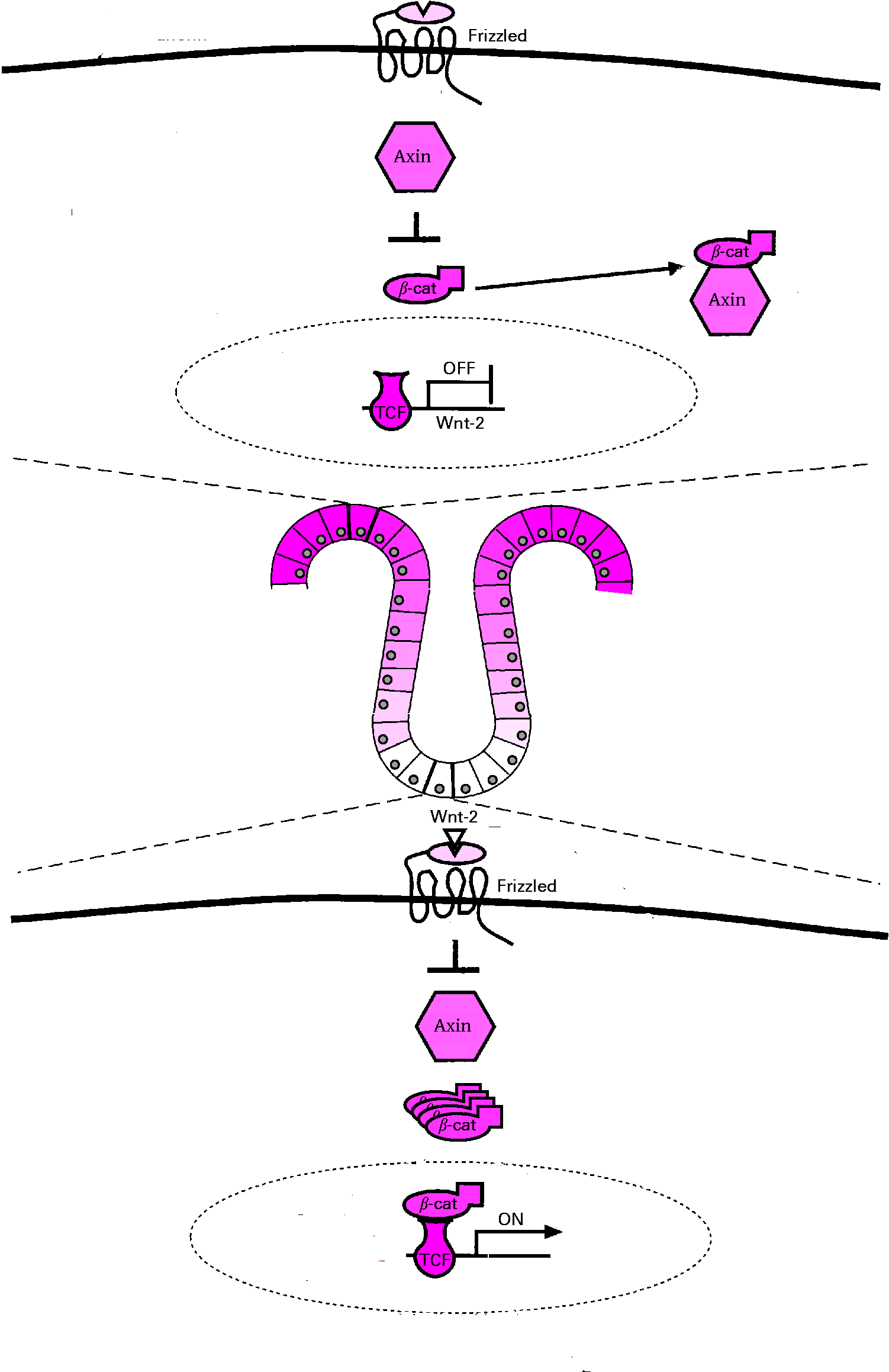## Intestinal renewal modelPi-calculus model combining intra-cellular signalling network based on Wnt pathway and inter-cellular interactions. Cellular decision to proliferate depends on the intracellular concentration of beta-catenin, multiplied by a factor molcycle = 0.0001 After a cell decides to proliferate, it completes a cycle at a constant rate cycle = 0.0014 A cell differentiates depending on the intracellular concentration of beta-catenin multiplied by a factor moldiff = 0.00001 Cells die at a constant rate die = 0.0001 (stem cells), die = 0 (differentiated cells) Depending on its position, a cell is stimulated with Wnt factors varying between pos = 0 and pos = 100